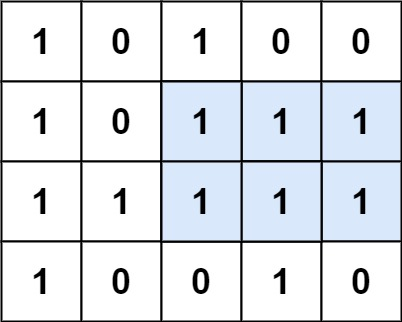## 85. Maximal Rectangle

Given a `rows x cols` binary `matrix` filled with `0`'s and `1`'s, find the largest rectangle containing only `1`'s and return its area.

Example 1:```Input: matrix = [["1","0","1","0","0"],["1","0","1","1","1"],["1","1","1","1","1"],["1","0","0","1","0"]]
Output: 6
Explanation: The maximal rectangle is shown in the above picture.
```

Example 2:

```Input: matrix = []
Output: 0
```

Example 3:

```Input: matrix = [["0"]]
Output: 0
```

Example 4:

```Input: matrix = [["1"]]
Output: 1
```

Example 5:

```Input: matrix = [["0","0"]]
Output: 0
```

Constraints:

• `rows == matrix.length`
• `cols == matrix.length`
• `0 <= row, cols <= 200`
• `matrix[i][j]` is `'0'` or `'1'`.

## Rust Solution

``````struct Solution;

impl Solution {
fn maximal_rectangle(matrix: Vec<Vec<char>>) -> i32 {
let n = matrix.len();
if n == 0 {
return 0;
}
let m = matrix.len();
let mut left = vec![0; m];
let mut right = vec![m; m];
let mut height = vec![0; m];
let mut res = 0;
for i in 0..n {
let mut l = 0;
let mut r = m;
for j in 0..m {
if matrix[i][j] == '1' {
height[j] += 1;
} else {
height[j] = 0;
}
}
for j in 0..m {
if matrix[i][j] == '1' {
left[j] = left[j].max(l);
} else {
left[j] = 0;
l = j + 1;
}
}
for j in (0..m).rev() {
if matrix[i][j] == '1' {
right[j] = right[j].min(r);
} else {
right[j] = m;
r = j;
}
}
for j in 0..m {
res = res.max((right[j] - left[j]) * height[j]);
}
}
res as i32
}
}

#[test]
fn test() {
let matrix = vec_vec_char![
['1', '0', '1', '0', '0'],
['1', '0', '1', '1', '1'],
['1', '1', '1', '1', '1'],
['1', '0', '0', '1', '0']
];
let res = 6;
assert_eq!(Solution::maximal_rectangle(matrix), res);
}
``````

Having problems with this solution? Click here to submit an issue on github.